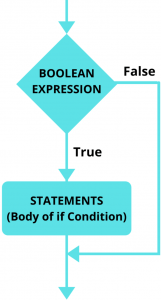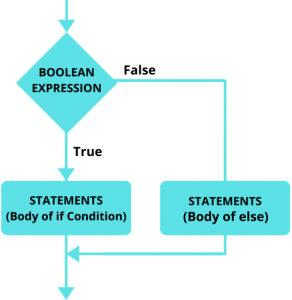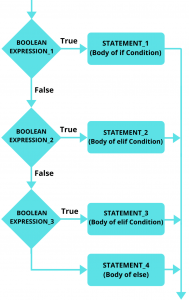Python if elif else: Decision making or conditional statement plays a major role in the world of computation.

Every program or software, we use has lots of decision-making statements that help the computer to understand or decide the flow of a program.

In this article, we are going to take a look at one of these decision-making statements which is the Python if elif else statement.

## Python if Statement

Python if statement consists of Boolean expression which evaluates as True or False.

Based on the result of this Boolean expression, the compiler decides whether to execute the set of code inside the if statement block or not.

Let’s understand this with the help of the Syntax of the if statement in Python.

### Syntax of Python if Statement

The syntax of the if statement is as follows:

```				```

if BOOLEAN_EXPRESSIONS:
STATEMENTS #This code gets executed when Boolean expression returns True
```
```

As you can see that we have first used the keyword “if”. Followed by that, we declared the Boolean expression.

So, if the Boolean expression evaluates to be True, then the indented statements inside the if block will get executed. Otherwise, the indented statements will not execute.

### Flowchart of Python if Statement

Here is the Flowchart of the if statement, which will help you in better understanding the flow of the program during the execution of the if statement.So, you can easily understand with the help of the above diagram that if the Boolean expression returns True, then the statements inside the Body of if Condition gets executed.

On the other hand, if the Boolean expression return False, then nothing gets executed.

Now, let’s understand the working of the if statement with the help of an example.

### Example of Python if Statement

Here is the basic example of if statement:

```				```
marks = 65

if marks > 44:
print("Student is Passed")
```
```
Output:
Student is Passed

As you can see in the above code that we have firstly initialized a variable “marks” with integer 65.

In the next line, we have used the if keyword followed by the Boolean expression.

This Boolean expression which is “marks > 44” returns True. Because the value of the marks variable is 65 which is greater than 44.

Since the Boolean expression returns True. So, the print statement gets executed.

Let’s see one more example of an if statement.

```				```
age = 16

if age > 18:
print("You are eligible for driving")
```
```

As you can see in the above code that the age variable which is 16 is not greater than 18. So, the Boolean expression return False.

As a result, the print statement doesn’t get executed.

## Python if else Statement

Now that you have seen the working of Python if statement. Let’s take a look at another most important conditional statement which is Python if else statement.

As we know that we use the if condition to execute the set of statements when the Boolean expression returns True.

However, what about when the Boolean expression returns False. Here comes the role of else statement.

We can use the else statement below the if statement block, to define the statements to be executed when the Boolean expression returns False.

So, let’s understand the basic syntax of Python if else statement.

### Syntax of Python if else Statement

Here is the basic syntax of the Python if else statement.

```				```
if BOOLEAN_EXPRESSION:
STATEMENT_1

else:
STATEMENT_2
```
```

As you can see from the above syntax of if else statement.

The STATEMENT_1 gets executed when the Boolean expression returns True. On the other hand, STATEMENT_2 gets executed when the Boolean expression returns False.

So, the set of code inside the if block gets executed when the Boolean expression returns True. Otherwise, the set of code inside the else block gets executed.

Let’s understand this in more detail with the help of a flowchart and example.

### Flowchart of Python if else Statement

Here is the flowchart of if else statement:With the help of the above diagram, you can understand that the statements inside the Body of if Condition gets executed when the Boolean expression returns True.

On the other hand, if the Boolean expression return False, then the statements inside the body of the else block get executed.

### Example of Python if else Statement

Here is the basic example of Python if else Statement:

```				```
age = 23

if age < 18:
print("You are not eligible for driving")

else:
print("You are eligible for driving")
```
```
Output:
You are eligible for driving

As you can understand with the help of the above example that since the age variable is equal to 23, which is not smaller than 18.

So, the Boolean expression returns False. As a result, the code inside the else block gets executed and we get the message “You are eligible for driving” on the screen.

## Python if elif else Statement

Here is the upgraded version of the Python if else statement, which is Python if elif else statement.

It is like a ladder of multiple if statement and single else statement. Where if any Boolean expression of if and elif statement returns True. Then, the rest of the Conditional Statements get ignored.

However, if none of the Boolean expressions of the if and elif statement returns true, then only the set of code inside the else block gets executed.

You will understand it much better with the help of Syntax, Flowchart, and Code example of if elif else statement.

### Syntax of Python if elif else Statement

The syntax of if elif else statement is as follows:

```				```
if BOOLEAN_EXPRESSION_1:
STATEMENT_1

elif BOOLEAN_EXPRESSION_2:
STATEMENT_2

elif BBOLEAN_EXPRESSION_3:
STATEMENT_3

else:
STATEMENT_4
```
```

If the BOOLEAN_EXPRESSION_1 returns True, then the STATEMENT_1 gets executed and the rest of the below code gets skipped.

However, if the BOOLEAN_EXPRESSION_1 returns False. Then the next block of code which is the elif statement gets executed.

Similarly, here also if BOOLEAN_EXPRESSION_2 returns True, then the STATEMENT_2 gets executed and the rest of the below code gets skipped.

However, if it returns False. Then the next block of code gets executed.

In case, none of the Boolean expressions return True. Then, the set of code inside the else block (STATEMENT_4) gets executed.

### Flowchart of Python if elif else Statement

Here is the flowchart of if elif else statement to explain the working of it.As you can see from the above diagram that if the BOOLEAN_EXPRESSION_1 returns True, then the STATEMENT_1 gets executed.

However, if it returns False. Then the BOOLEAN_EXPRESSION_2 gets evaluated.

Here also if the BOOLEAN_EXPRESSION_2 returns True, then the STATEMENT_2 gets executed.

On the other hand, if it returns False. Then the BOOLEAN_EXPRESSION_3 gets evaluated.

Similar is the case with BOOLEAN_EXPRESSION_3. Here also the Boolean expression gets evaluated.

So, if the BOOLEAN_EXPRESSION_3 returns True, then the STATEMENT_3 gets executed.

Otherwise, the set of code inside the else block will get executed.

Best Course

-> Best Course for learning Python: Python for Everybody Specialization

### Example of Python if elif else Statement

Here is the basic example of Python if elif else statement:

```				```
marks = 65

if marks >= 90:
print("Excellent")

elif marks >= 80:
print("Very Good")

elif marks >= 70:
print("Good")

elif marks >= 60:
print("Average")

elif marks >= 44:
print("Need Improvement")

else:
print("Failed")
```
```
Output:
Average

As you can see in the above code, that the value of variable marks is 65.

Since 65 is not greater than 90. So, the print statement doesn’t get executed.

Similarly, In the elif blocks, the Boolean expression returns False.

However, In one of the elif statements which is “marks >= 65”, the Boolean expression returns True.

As a result, the print statement gets executed and the message “Average” gets printed on the screen, and the rest of the below code gets skipped.

## Python Nested if Statement

Python nested if statement is a set of if else statements inside another if else statement.

So, inside if or else block, we will be using another if else statement.

You will understand it much better with the help of the below example.

```				```
amount = 700

if amount > 500:
print("You are eligible for Shopping")

if amount > 1000:

else:
print("You can buy 5 products only")

else:
print("You are not eligible for Shopping")
```
```
Output:
You are eligible for Shopping
You can buy 5 products only

As you can see in the above code that inside the if statement, we have used another if else block of code.

In the above code, the value of the variable “amount” is 700. So, In the if condition, the Boolean expression which is “amount > 500” returns True. As a result, the print statement gets executed.

Then inside this, if statement, we get another if else block, where the Boolean expression of if statement which is “amount > 1000” returns False.

As a result, else statement gets executed with the print function inside it.

Let’s see one more example of a nested if else statement.

```				```
amount = 700

if amount > 500:
print("You are eligible for Shopping")

if amount > 800:

if amount > 700:

else:
print("You can buy only 1 product")
```
```
Output:
You are eligible for Shopping
You can buy only 1 product

Here the Boolean expression of the first if statement returns True. As a result, the print statement with the message “You are eligible for shopping” gets executed.

Then inside this, if statement block, there are two if statements and one else statement.

As the value of the amount variable is not greater than 800 and 700, the Boolean expression of these if statements return False.

So, the else statement gets executed and we get the message “You can buy only one product” on our screen.

## Python if else Statement in One Line

Now, we are going to take a look at the compressed version of the if else statement which is also called as Shorthand if else statement.

In Shorthand if else statement, if the Boolean expression of the if statement returns True, then the code before the if statement gets executed. Otherwise, the code after the else keyword gets executed.

```				```
level = 8

print("Pro" if level > 7 else "Noob")
```
```
Output:
Pro

As you can see in the above code that the variable named “level” is equal to 8.

So, In the next line, inside the print function, we used the Shorthand if else statement, where we checked whether “level > 7” or not.

Since the value of the level is greater than 7. So, it returns True and we get the message “Pro” on our screen.

Hope you like the article on Python if elif else statement. We also have an article on Python for Loop and while Loop, which you must take a look at.